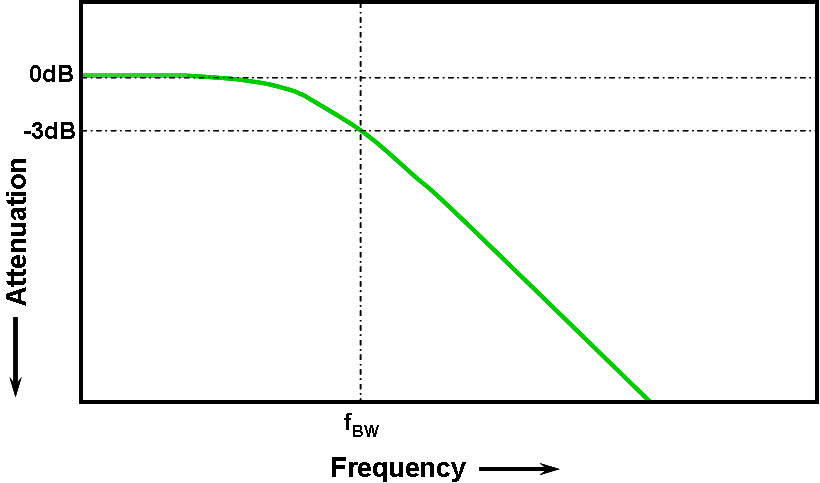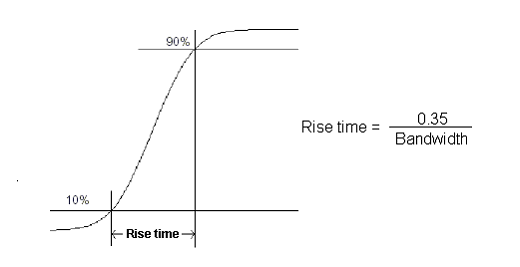Lilliput Electronics (USA) Inc.

130 Commerce Way, Walnut CA 91789 , USA

You are here: Home >Technical Press >Content
Why is the measured amplitude less than the real value?
- 6/25/2017 10:00:00 AM -

Try a small test. Use your 100 MHz oscilloscope to measure a 100MHz, 3.3V amplitude waveform. The measured amplitude isn’t accurate. This issue refers to the bandwidth of oscilloscope.

What is bandwidth?

Bandwidth is an essential parameter for an oscilloscope, but what is bandwidth? Bandwidth refers to the analogue bandwidth of the analogue front end of the oscilloscope, and directly determines the oscilloscope’s signal measurement capabilities. Specifically, the oscilloscope bandwidth is the highest frequency when the amplitude of the sine wave measured by the oscilloscope is not lower than the 3dB amplitude of the true sine wave signal (i.e. 70.7% of the true signal amplitude), also known as -3dB cut off frequency point. As the signal frequency increases, the oscilloscope's ability to accurately display the signal level will reduce.When the measured sine wave frequency is equal to the bandwidth of the oscilloscope (oscilloscope amplifier is for the Gaussian response), we can see that the measurement error is about 30%. If the measurement error is required to be 3%, the frequency of the measured signal should be much lower than the oscilloscope bandwidth. For example, using a 100MHz oscilloscope to measure a 100MHz, 1Vpp, sine wave signal, the measurements will be 100MHz, 0.707Vpp, sine wave waveform. This is only the case for a sine wave since most waveforms are much more complex than a sine wave they will contain higher frequencies. So in order to achieve a certain measurement accuracy, we use the oscilloscopes common law that is commonly referred to as 5 times the standard:

The required bandwidth of the oscilloscope = the highest frequency of the measured signal * 5

2. Select the bandwidth correctly

Complex signals in a waveform are formed by a variety of different harmonic sine wave signals, and the bandwidth of these harmonics may be very wide. When the bandwidth is not high enough, the harmonic components will not be effectively amplified (blocked or attenuated), which may cause amplitude distortion, edge loss, loss of detail data, etc. The signal characteristics such as bells and tones, etc. will have no reference value.

So, for different frequency signal measurements, the correct bandwidth is very important. When measuring high frequency signals, such as measuring a 27MHz crystal, you should use the full bandwidth measurement.

If the bandwidth limit is enabled, that is, the bandwidth limit is set to 20MHz, the crystal waveform will be distorted and the measurement will be of no value. When measuring low-frequency signals, you should set the bandwidth limit to enable the high-frequency signal interference filter, this is so the signal shows more clearly.

3. Bandwidth and rise time

With regards to bandwidth, the rise time cannot be ignored. The rise time is usually defined as the time at which the signal amplitude changes from 10% of the maximum steady value to 90%.The bandwidth of the oscilloscope can directly show the minimum rise time of the signal. The rise time of the oscilloscope system can be evaluated from the specified bandwidth. You can use formulate: RT (rising time) = 0.35 / BW (bandwidth) (oscilloscope below 1GHz) to calculate.

Where 0.35 is the scale factor between the oscilloscope bandwidth and the rise time (10% -90% rise time in the first order Gaussian model). According to the above formula, if the bandwidth of the oscilloscope is 200MHz, can calculate RT = 1.75ns, that is, the minimum observable rise time.